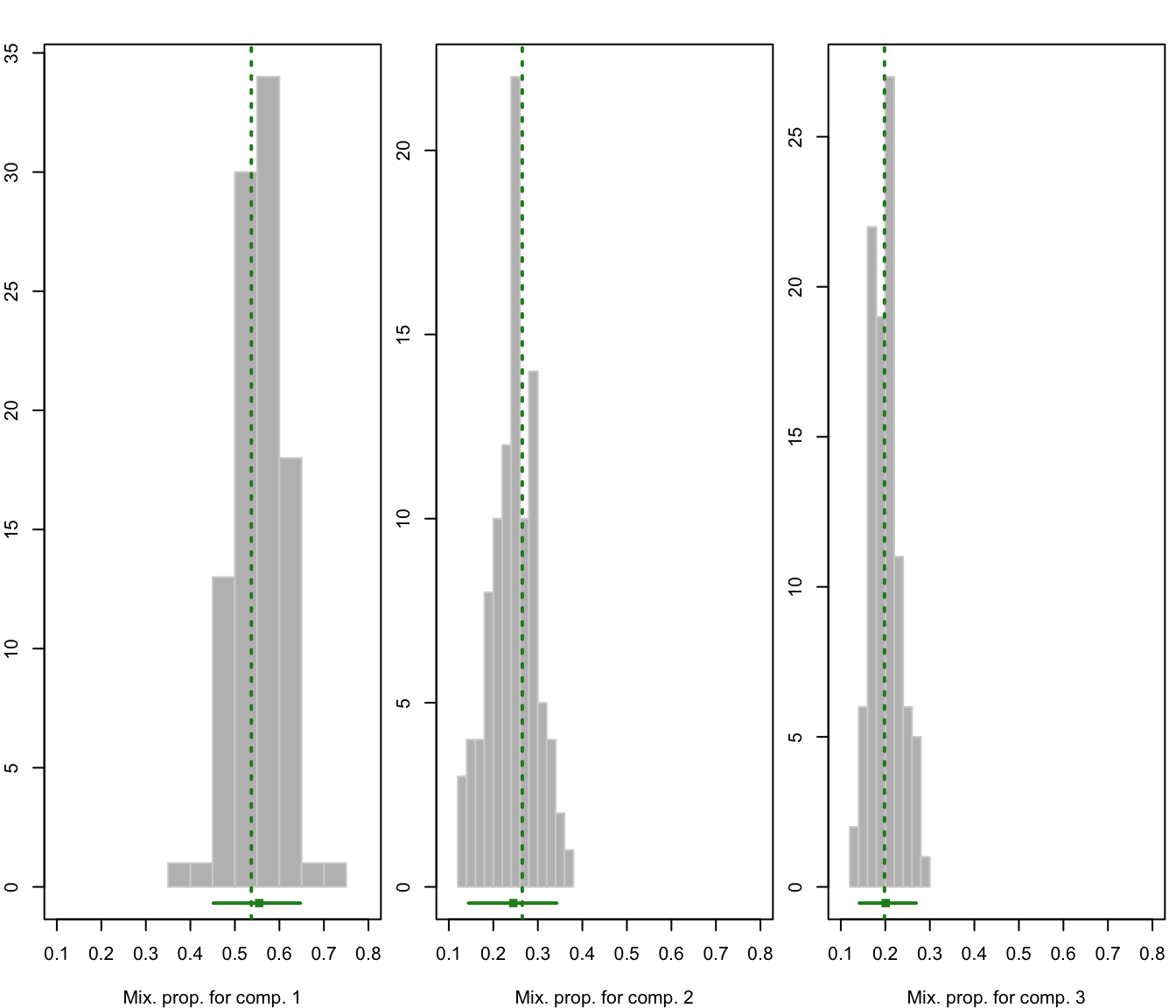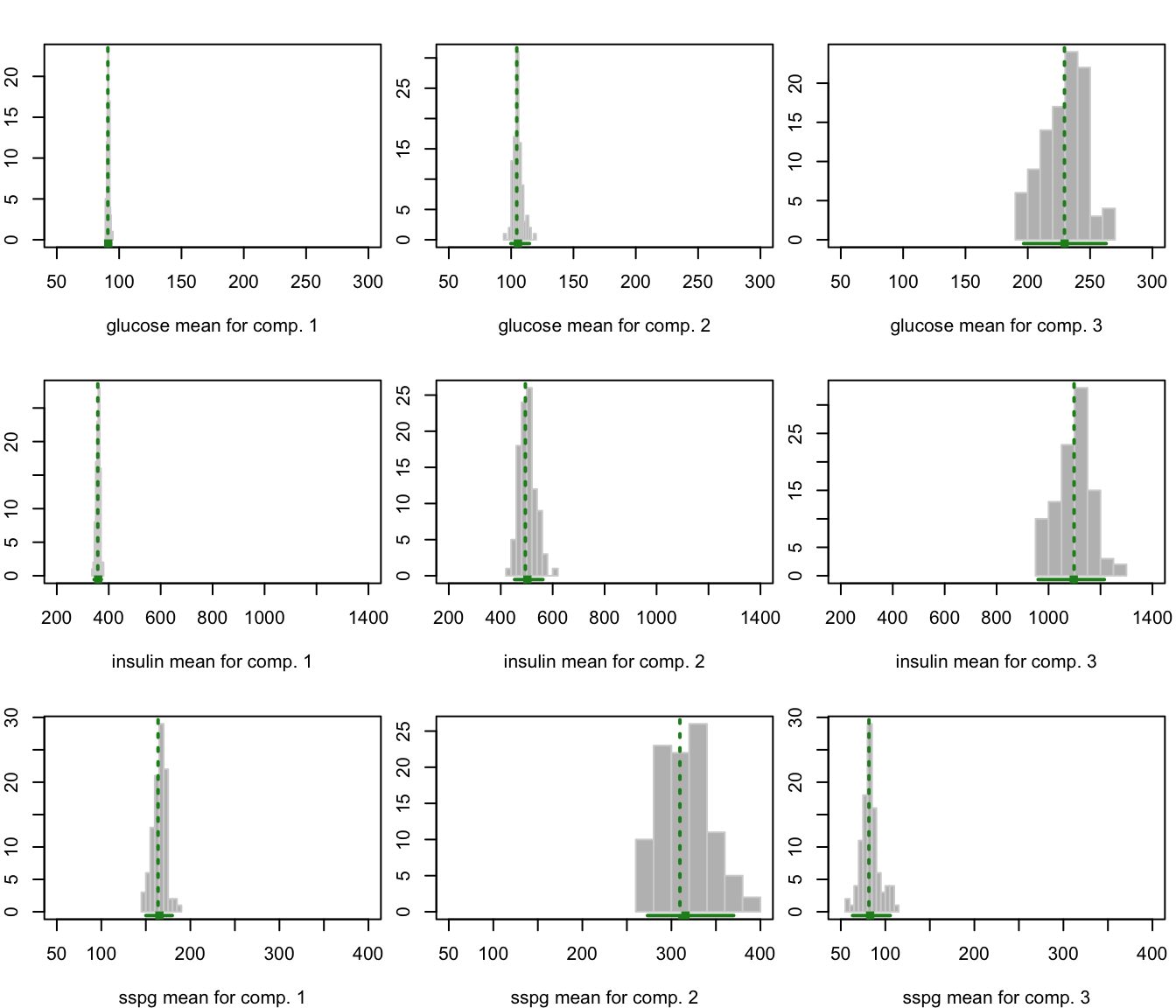Plots the bootstrap distribution of parameters as returned by the MclustBootstrap function.

# S3 method for MclustBootstrap
plot(x, what = c("pro", "mean", "var"),
show.parest = TRUE, show.confint = TRUE,
hist.col = "grey", hist.border = "lightgrey", breaks = "Sturges",
col = "forestgreen", lwd = 2, lty = 3,
xlab = NULL, xlim = NULL, ylim = NULL, ...)

Arguments

x

Object returned by MclustBootstrap.

what

Character string specifying if mixing proportions ("pro"), component means ("mean") or component variances ("var") should be drawn.

show.parest

A logical specifying if the parameter estimate should be drawn as vertical line.

show.confint

A logical specifying if the resampling-based confidence interval should be drawn at the bottom of the graph. Confidence level can be provided as further argument conf.level; see summary.MclustBootstrap.

hist.col

The color to be used to fill the bars of the histograms.

hist.border

The color of the border around the bars of the histograms.

breaks

See the argument in function hist.

col, lwd, lty

The color, line width and line type to be used to represent the estimated parameters and confidence intervals.

xlab

Optional label for the horizontal axis.

xlim, ylim

A two-values vector of axis range for, respectively, horizontal and vertical axis.

...

Other graphics parameters.

Value

A plot for each variable/component of the selected parameters.

Examples

# \donttest{
data(diabetes)
X <- diabetes[,-1]
modClust <- Mclust(X, G = 3, modelNames = "VVV")
bootClust <- MclustBootstrap(modClust, nboot = 99)
par(mfrow = c(1,3), mar = c(4,2,2,0.5))
plot(bootClust, what = "pro")par(mfrow = c(3,3), mar = c(4,2,2,0.5))
plot(bootClust, what = "mean")# }